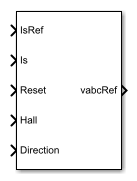# BLDC Current Controller

Discrete-time Brushless DC Motor current PI controller

• Library:
• Simscape / Electrical / Control / BLDC Control

•## Description

The BLDC Current Controller block uses this algorithm to control current in a DC brushless motor.### Equations

The BLDC Current Controller produces the duty cycle for a BLDC block by implementing proportional-integral (PI) current control using this equation.

`$\text{D}=\left({K}_{p}+{K}_{i}\frac{{T}_{s}z}{z-1}\right)\left({I}_{s_ref}-{I}_{s}\right)$`

Where:

• D is the duty cycle.

• Kp is the proportional gain.

• Ki is the integral gain.

• Ts is the time period.

• Is_ref is the reference current.

• Is is the measured current.

• Gzc is the zero cancellation polynomial.

The closed-loop transfer function for the PI control algorithm yields a zero that can be cancelled by using zero-cancellation in the feedforward path. The zero-cancellation transfer function in discrete-time is:

`${G}_{ZC}\left(z\right)=\frac{\frac{{T}_{s}{K}_{i}}{{K}_{p}}}{z+\left(\frac{{T}_{s}-\frac{{K}_{p}}{{K}_{i}}}{\frac{{K}_{p}}{{K}_{i}}}\right)}.$`

The block obtains control signals for the three phases by multiplying the duty cycle by the commutation signals. The resulting three control signals are normalized over the interval [-1, 1].

## Ports

### Input

expand all

Reference current for control.

Data Types: `single` | `double`

Actual current.

Data Types: `single` | `double`

External reset signal (rising edge) for the integrator.

Data Types: `single` | `double`

Hall sensor data.

Data Types: `single` | `double`

Direction of motor rotation.

Data Types: `single` | `double`

### Output

expand all

Reference voltage for the a-, b-, and c-phases.

Data Types: `single` | `double`

## Parameters

expand all

Proportional gain, Kp, of the controller.

Integral gain, Ki, of the controller.

Anti-windup gain, Kaw, of the controller.

Time, in s, between consecutive block executions. During execution, the block produces outputs and, if appropriate, updates its internal state. For more information, see What Is Sample Time? and Specify Sample Time.

If this block is inside a triggered subsystem, inherit the sample time by setting this parameter to `-1`. If this block is in a continuous variable-step model, specify the sample time explicitly using a positive scalar.

#### Dependencies

If you set Sample time (-1 for inherited) to `-1` and select the Enable zero cancellation option, the Discretization sample time parameter becomes visible.

Time, in s, between consecutive discretizations. Discretization is required for zero cancellation.

#### Dependencies

This parameter is only visible when both these conditions are met:

• is set to `-1`.

• Enable zero cancellation is selected.

Option to use zero cancellation on the feedforward path.

#### Dependencies

If you select the Enable zero cancellation option and set Sample time (-1 for inherited) to `-1`, the Discretization sample time parameter becomes visible.

 Stirban, A., I. Boldea, and G. D. Andreescu. "Motion-Sensorless Control of BLDC-PM Motor With Offline FEM-Information-Assisted Position and Speed Observer." IEEE Transactions on Industry Applications. 48, no. 6 (2012): 1950-1958.